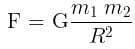NEET  >  Test: Universal Law of Gravitation

# Test: Universal Law of Gravitation

Test Description

## 10 Questions MCQ Test | Test: Universal Law of Gravitation

Test: Universal Law of Gravitation for NEET 2023 is part of NEET preparation. The Test: Universal Law of Gravitation questions and answers have been prepared according to the NEET exam syllabus.The Test: Universal Law of Gravitation MCQs are made for NEET 2023 Exam. Find important definitions, questions, notes, meanings, examples, exercises, MCQs and online tests for Test: Universal Law of Gravitation below.
Solutions of Test: Universal Law of Gravitation questions in English are available as part of our course for NEET & Test: Universal Law of Gravitation solutions in Hindi for NEET course. Download more important topics, notes, lectures and mock test series for NEET Exam by signing up for free. Attempt Test: Universal Law of Gravitation | 10 questions in 10 minutes | Mock test for NEET preparation | Free important questions MCQ to study for NEET Exam | Download free PDF with solutions
 1 Crore+ students have signed up on EduRev. Have you?
Test: Universal Law of Gravitation - Question 1

### SI unit of gravitational constant is __________.

Detailed Solution for Test: Universal Law of Gravitation - Question 1

We know that:Thus we get, [G] = [F.R2 / m2 ] = [F] x L2 x M-2 = [F] M-2 L= N kg-2 m2

Test: Universal Law of Gravitation - Question 2

### The dimensions of gravitational constant G is:

Detailed Solution for Test: Universal Law of Gravitation - Question 2

From Newton’s law of gravitation,Since, Force (F) = Mass × Acceleration = M × [LT-2]
∴ The dimensional formula of force = M1 L1 T-2

⇒ Gravitational Constant (G) = F × r2 × [m1m2]-1
Or, G = [M1 L1 T-2] × [L]2 × [M]-2 = [M-1 L3 T-2].

Therefore, the gravitational constant is dimensionally represented as [L][M]-1 [T]-2.

Test: Universal Law of Gravitation - Question 3

### The tides are formed due to:

Detailed Solution for Test: Universal Law of Gravitation - Question 3

Tides refer to the rise and fall of our oceans' surfaces. It is caused by the attractive forces of the Moon and Sun's gravitational fields as well as the centrifugal force due to the Earth's spin.

Test: Universal Law of Gravitation - Question 4

Two bodies with same mass “m” separated by a distance “r” exert a gravitational force of F on each other. Suppose the distance between them is doubled and the force becomes F’. The ratio of two forces is

Detailed Solution for Test: Universal Law of Gravitation - Question 4

We know that the force of gravitation is inversely proportional to square of the distance between the two bodies,

i.e. F∝ r-2

Hence, when the distance between them will be doubled, the force will be reduced by 4 times

So, the ratio will be 4:1

Test: Universal Law of Gravitation - Question 5

The mass of the body on moon is 40kg, what is the weight on the earth.

Detailed Solution for Test: Universal Law of Gravitation - Question 5

Weight = Mass * Accelertion due to gravity = 40 * 9.8 = 392 N

Test: Universal Law of Gravitation - Question 6

What is the value of G in cgs system of units?

Detailed Solution for Test: Universal Law of Gravitation - Question 6

The value of G is universally constant = 6.67 × 10-8 dyne.cm2/g2

We know:
1 dyne = 10-5 N
1 cm = 10-2 m
1 g = 10-3 kg

⇒ 6.67 × 10-8 × ( 10-5) .(10-2)2/(10-3)2 Nm2/kg2 = 6.67 × 10-8 { 10-5× 10-4 /10-6} Nm2/kg2
= 6.67 × 10-8 × 10-3 N.m2/kg2= 6.67× 10-11 Nm2/kg2

Test: Universal Law of Gravitation - Question 7

Gravitation follows:

Detailed Solution for Test: Universal Law of Gravitation - Question 7
• Gravitational force strictly follows Newton’s Third Law of motion i.e. for every action force there is an equal and opposite reaction force.
• Gravitational forces add up like vectors i.e. they obey the principle of superposition.
Test: Universal Law of Gravitation - Question 8

G is a universal gravitational constant, its value is:

Detailed Solution for Test: Universal Law of Gravitation - Question 8

We know that: [g] = [G.m.m / r.r]
Since the unit of G must be same as the unit of g.r.r / m.m  i.e. Nm2 /kg2

Also, we know that G is very very less than 1 as the gravitational force is a very weak force.

Test: Universal Law of Gravitation - Question 9

In orbit, objects inside a spacecraft feel weightless:

Detailed Solution for Test: Universal Law of Gravitation - Question 9
• Astronauts float around in space because there is no gravity in space.
• Astronauts are so far from the Earth that gravity is so small. This is why NASA calls it microgravity.
Test: Universal Law of Gravitation - Question 10

What pulls a ball back to earth?

Detailed Solution for Test: Universal Law of Gravitation - Question 10

The gravitational force of the earth acts on every object having mass. When a ball is thrown upwards the gravitational force of the earth acts against the velocity of the object and pulls the ball downwards, i.e, towards itself.

Thus, gravity pulls a ball back to earth.

Information about Test: Universal Law of Gravitation Page
In this test you can find the Exam questions for Test: Universal Law of Gravitation solved & explained in the simplest way possible. Besides giving Questions and answers for Test: Universal Law of Gravitation, EduRev gives you an ample number of Online tests for practice

### How to Prepare for NEET

Read our guide to prepare for NEET which is created by Toppers & the best Teachers(Scan QR code)